# Multi-Tapped Toroidal Inductor questions

Hi,
The thing is that i want to wind a multi-tapped toroid inductor for the this circuit (see picture at the bottom)
Using an MPP core.

What i am confused about is this.
On the following table you can see that when the permeability of an MPP core increases
the high frequencies it can handle will decrease, is this correct?
This must have an impact on the Q factor as well, because i would need more wire on a lower perm core, so the overall resistance goes up.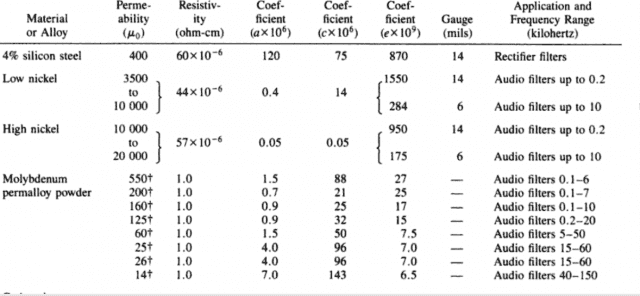What i know so far is..
To achieve best results, all tapped windings should be evenly distributed 360º around the core, and all taps should exit the core at nearly the same point.

And then we have reverse winding and double reverse winding techniques to limit capacitive losses?
must be the same as progressive winding i guess?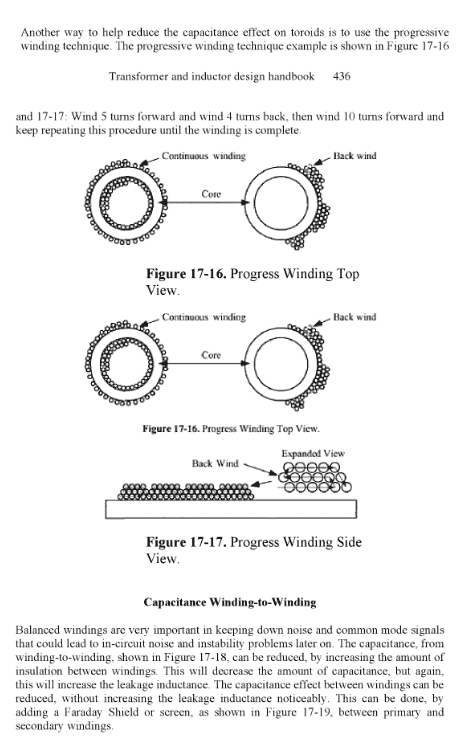I would like to know if i can measure the capacitive losses with my LCR meter?
Is this simply measured like this:
Measuring capacitance from tap 1 to all the other taps?
I am pretty new to this, but i know how to calculate the turns i need and so on, but any tips or information on this would be appreciated a lot.

Kind regards.

Schematic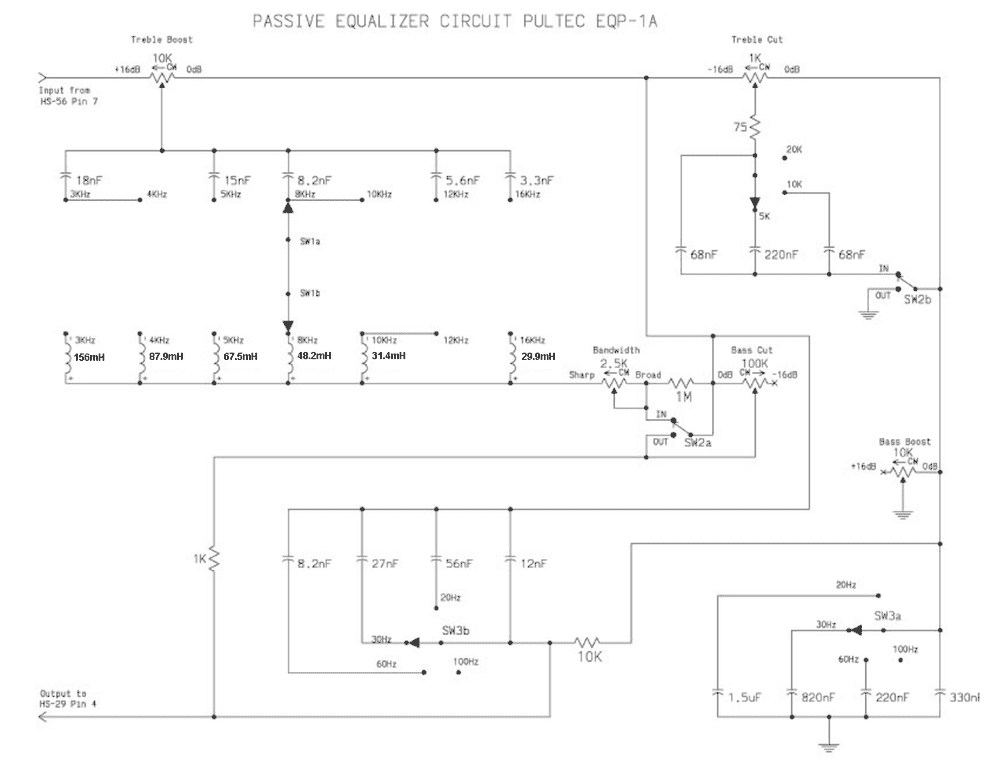Baluncore
On the following table you can see that when the permeability of an MPP core increases the high frequencies it can handle will decrease, is this correct?
This must have an impact on the Q factor as well, because i would need more wire on a lower perm core, so the overall resistance goes up.
Higher frequencies require thinner laminations, or smaller particles in the core because the magnetic field must penetrate the particle and then reverse for each cycle, without high losses. Smaller particles require the same insulation thickness, so the proportion of magnetic material falls.

The Q factor is a function of the reactance to resistance ratio. At higher frequencies the reactance of an inductor rises, XL = 2·π·f·L; which should cancel most of the higher wire resistance effect on Q.

I would like to know if i can measure the capacitive losses with my LCR meter?
You cannot separate the capacitive losses from the inductive losses, but you can determine the capacitance in parallel with the inductive winding.
To do that you measure the L and series R at two different frequencies with your meter. If the inductance readings differ you can solve for the capacitance in parallel with the inductor. You know that the inductance reported has an equivalent inductive reactance, and that reactance is the parallel sum of inductive and capacitive reactances at that frequency. You know that inductive reactance is XL = 2·π·f·L; and capacitive reactance XC = -1/(2·π·f·C); so capacitance lowers the effective inductance at higher frequencies. Since it is a parallel circuit you may find it easier to solve using the admittance = reciprocal reactance. You can calculate the self resonant frequency from the parallel L and C values.

Hi Baluncore,
Thank you very much for the reply.
Very good explanation you provided, the best permeability for this circuit using an MPP core would be 125u then.
I am still pretty new to this, the LCR meter i am using is called DE 5000.
The test frequencies it can provide is 100 / 120 / 1k / 10k / 100kHz
So what i understand so far is that in reality the inductor is looking like this.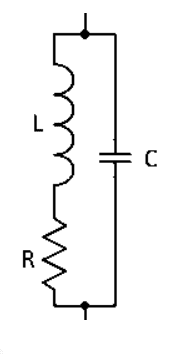So when i am done with the first turns for the 29.9mH i should then cut a lille of the insulation off to make a measurement of the L, lets just assume this is 29.9mH spot on.
I should then make a measurement of the R, lets say this is 10 ohms, i should then make a measurement again using a higher test frequency and see how the values differ, or is this just plain wrong?
Sorry i am very new to this, so if you could provide me with a step by step guide to do this, it would be appreciated a lot.

Thanks again.

Kind regards.

Baluncore
You are overthinking the problem.
Count the turns, n, while winding one layer onto the core. Measure the inductance, L, with your meter at a frequency near operating frequency. Calculate the value of AL for the core by AL = L / n²; That gives you a real number for your particular core.

Then calculate the number of turns needed for 29.9 mH by n = √( L / AL ). Wind that coil, but keep some spare wire. Measure the inductance near the operating frequency to check your count. Add or subtract turns to bring the value of L to 29.9 mH.

The number of turns is the number of times the wire passes through the hole in a toroidal core. The external lead length, or dress, has little effect on inductance.

If you need to trim it later, it is easier to pad the capacitors than adjust the inductor.
If you must trim a tapped inductor you do not need to rewind the coils. Connect a short wire to the tap and wind it forwards or backwards to effectively add or subtract turns to that tap.

You will not know what you are doing until you have done it.
Wind the first inductor now. Extend it to other range taps later.
Try not to start multiple threads on the same subject.

•anorlunda
Svein
I created an equalizer once based on your principle. I did not, however, want to use inductors (big, heavy and prone to pick up magnetic noise), so I replaced the inductors in the schematic with gyrators. The equalizer worked really well...

You are overthinking the problem.
Count the turns, n, while winding one layer onto the core. Measure the inductance, L, with your meter at a frequency near operating frequency. Calculate the value of AL for the core by AL = L / n²; That gives you a real number for your particular core.

Then calculate the number of turns needed for 29.9 mH by n = √( L / AL ). Wind that coil, but keep some spare wire. Measure the inductance near the operating frequency to check your count. Add or subtract turns to bring the value of L to 29.9 mH.

The number of turns is the number of times the wire passes through the hole in a toroidal core. The external lead length, or dress, has little effect on inductance.

If you need to trim it later, it is easier to pad the capacitors than adjust the inductor.
If you must trim a tapped inductor you do not need to rewind the coils. Connect a short wire to the tap and wind it forwards or backwards to effectively add or subtract turns to that tap.

You will not know what you are doing until you have done it.
Wind the first inductor now. Extend it to other range taps later.
Try not to start multiple threads on the same subject.

Okay i see thanks for the tips, and thank you for taking the time to clear this up for me.

Kind regards

Baluncore
Once you have wound an inductor you can estimate the parallel capacitance.
Use your meter to measure the inductance at two different frequencies.
Readings are; L1 at f1; and L2 at f2.
If L1 is different to L2 then measurable parallel capacitance is present.

To get the actual inductance and estimate parallel capacitance.
Compute angular frequencies.
w1 = TwoPi * f1
w2 = TwoPi * f2

y1 = 1 / ( L1 * w1 )
y2 = 1 / ( L2 * w2 )

Compute actual L and parallel C.
L = ( w2/w1 - w1/w2 ) / ( w2*y1 - w1*y2 )
C = ( y1 - 1 / ( L * w1 ) ) / w1

Compute a self-resonant frequency for the inductor.
F = 1 / ( TwoPi * Sqr( L * C ) )

Thank you!
I think i get it now, i should measure L at lowest test frequency possible, where i would get a reading of course.
this is L1, f1.
I should then measure L at highest frequency possible.
The reading i get at a higher frequency would be lower, because the parasitic capacitance is reducing L.
this is of course L2, f2.
Armed with the two, i can now get the SRF and solve C using the formulas.
I have not got the core yet, so i cant make the coil, i could maybe measure and calculate this for each layer and use the progressive winding technique to reduce the capacitance, i will try out different methods, as long as i have an estimate that´s fine.

Thanks again,
Kind regards.

Baluncore
... i should measure L at lowest test frequency possible, where i would get a reading of course ...
I should then measure L at highest frequency possible ...
Going to extremes is a mistake. An inductance meter might operated from 10 Hz to 100kHz, both outside the band of interest. Inductance bridge frequency steps are usually factors of 10, which is more than sufficient to estimate parallel capacitance.

First measure L close to the frequency of operation, and then at one frequency step below. That will give more realistic results because it reduces the effect of core permeability variation with frequency.

Okay i see.
This is what i have available on the LCR meter100Hz/120Hz/1kHz/10kHz /100kHz
I do actually have a function signal generator 1Hz-500kHz and a standard DMM, but i would only be able to measure 60-50Hz AC on this DMM, so that would be insufficient, it would need to be 100kHz AC as i understand.

One way could maybe be set the signal generator in parallel with the inductor, then make a modified RF probe with x100 larger capacitors, and put that in parallel as well, measure low voltage DC, sweep the signal generator, and find the frequency where the signal is largest on the DMM.
That way i should be able to find the SRF.

Then again i think i will try out with the LCR meter first, and see if i can figure this out,
I do not have the components to make this simple circuit right now, but i would maybe try it out in the future.

Schematic probe.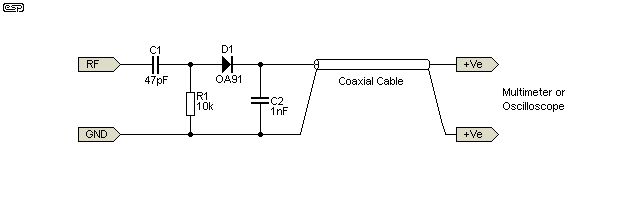Baluncore
The LCR meter should do the job.

Finding the centre frequency by maximum amplitude is difficult because the top of the resonance is flat and you can never be sure which way to search. You will get a more accurate result by using the signal generator to drive the LC resonant circuit through a 1k0 resistor, while looking for zero phase shift across the resistor with a dual trace oscilloscope. That way you will know which side of the resonance you are on during the search.

This is true, great idea.
Wish i had an oscilloscope, i should get one in the future, that would be more accurate and easier :)

Would it be safe to assume the goal is to replicate the Pultec EQP-1? (Based on teh name on the schematic...)

So then I would also assume the idea is to replicate the sound from this particular piece of vintage audio gear?

If that is the case then as you likely also know that the pieces of gear that achieve legendary status among their fans etc is mostly due to the magic they impart on the audio due to their characteristic non linearities. Are you also building the valve gain stage?

https://vintageking.com/blog/2017/11/pultec-bf/
Normally here all the things you do with reducing interwinding capacitance and choosing linear core materials, esp more modern materials, may actually not be what you want, that may be part of the source of its character. Do you have one to copy? Can you find images of how it was built? Do you know what core materials are used in it? Maybe nonlinear core materials (ie permeability changes amplitude) is where its character comes from (like soft saturation of valve amplifier output transformers).

Perhaps one approach to winding style etc, is copy the original if you can find images etc?

I've always had a soft spot for old analog audio gear, more on the analog synth side though, the moog ladder filter for example.

Hi essenmein,
Yes this is for a Pultec EQP-1A replica, i do also have a very soft spot for vintage audio gear
I love the sound on some of the original units, but looking for tweaks that would make it even better.

I do have some info on the original coil, but i have never had one in my hand.
The original coil with an MPP core labeled, EWC 140 had some other mH values because the caps had some other values as well (info from vintagewindings.com)
Outside diameter: 23mm
Inside Diameter: 14mm
Height: 8mm
Turns in total around 1300 (info from the 5 tap version)

Heard a lot of rumors about the coil used, of course these old engineers knew what they were doing, but they also had a budget, and i think a better coil can be made now, using a bigger core, and with a higher permeability so the number of turns would be reduced a little, saturation in an inductor should be avoided because it will
result in a ringing sound.

The transformers do have a great sound i have used the original input transformer Triad HS-56V and the Original interstage HS-29

The original Peerless 217-D output transformer was very hard to find and gave up, so used a Cinemag 217-D
This one should be identical to the original design.

The power transformer is a Hammond 370BX same specs as the original
i dont want old power transformers.

NOS Telefunken for the for the AX7
Rest is NOS RCA tubes.

The electrolytic caps are also new Sprague Atoms hiding inside some cans on the back.
The brass dome should be the cover for the inductor.
Everything is pretty much like the original, its for recordings in a studio so i want the best out of it :)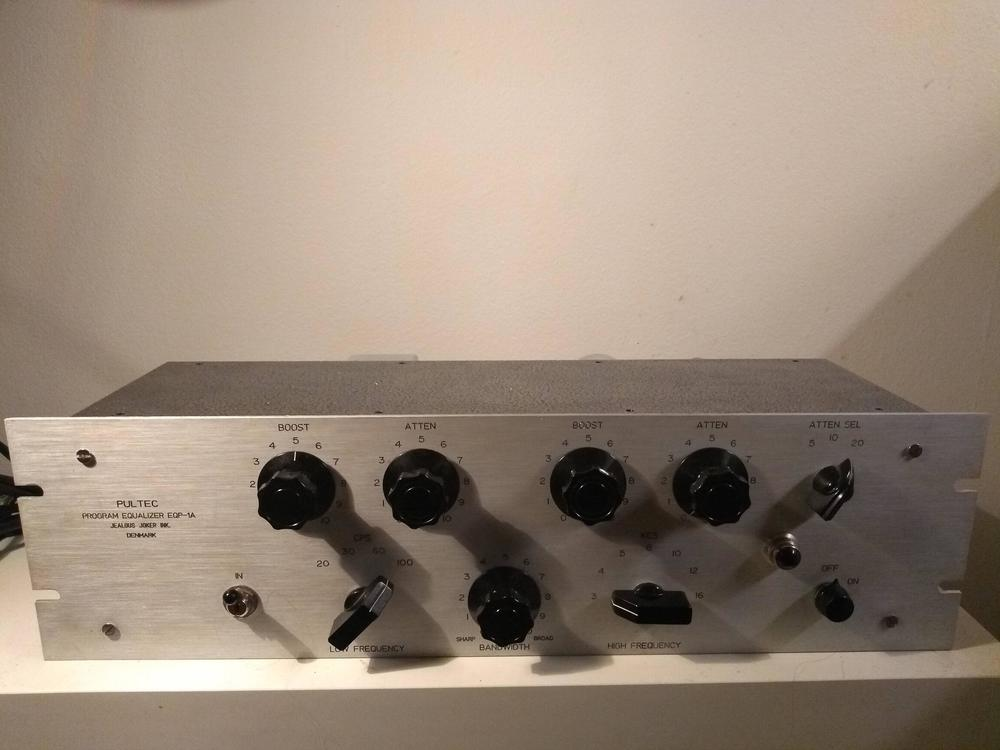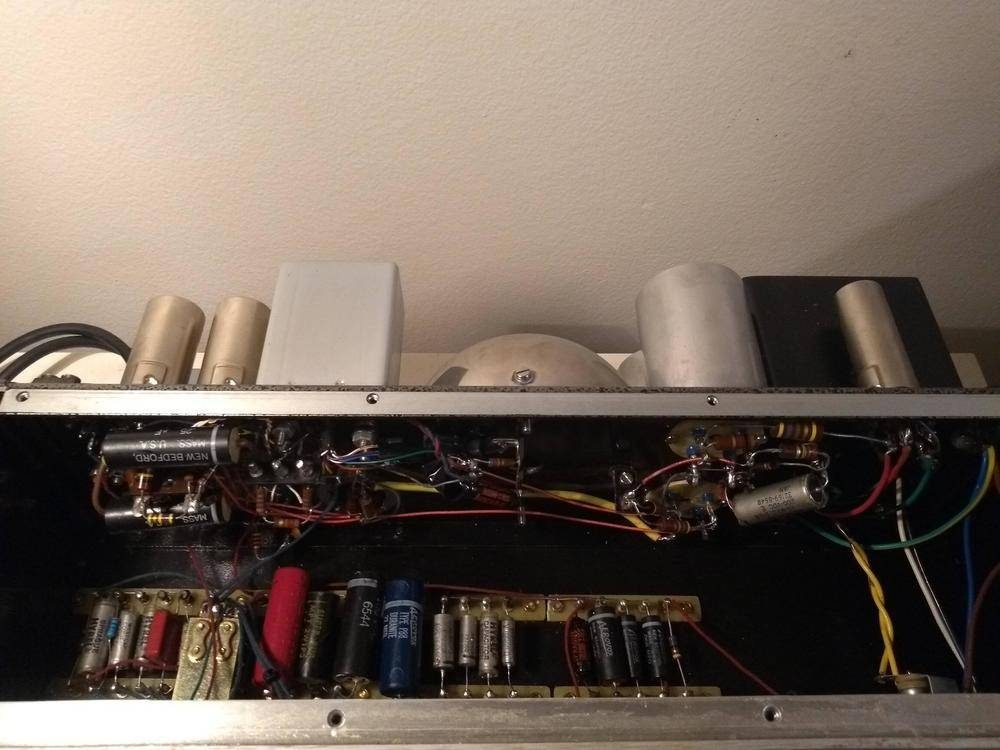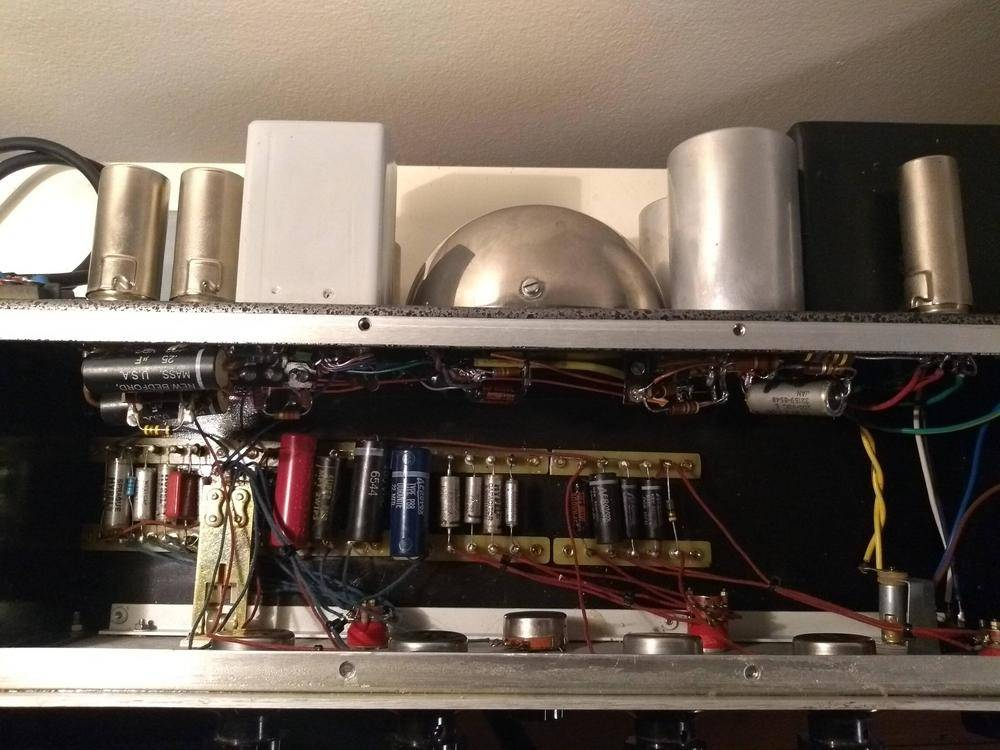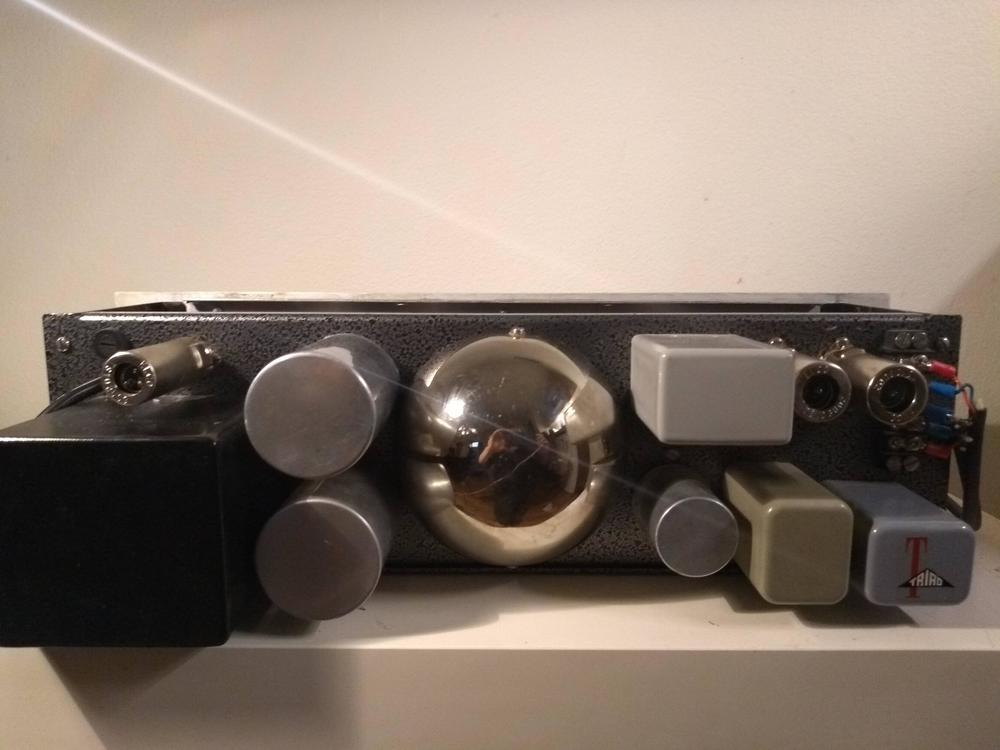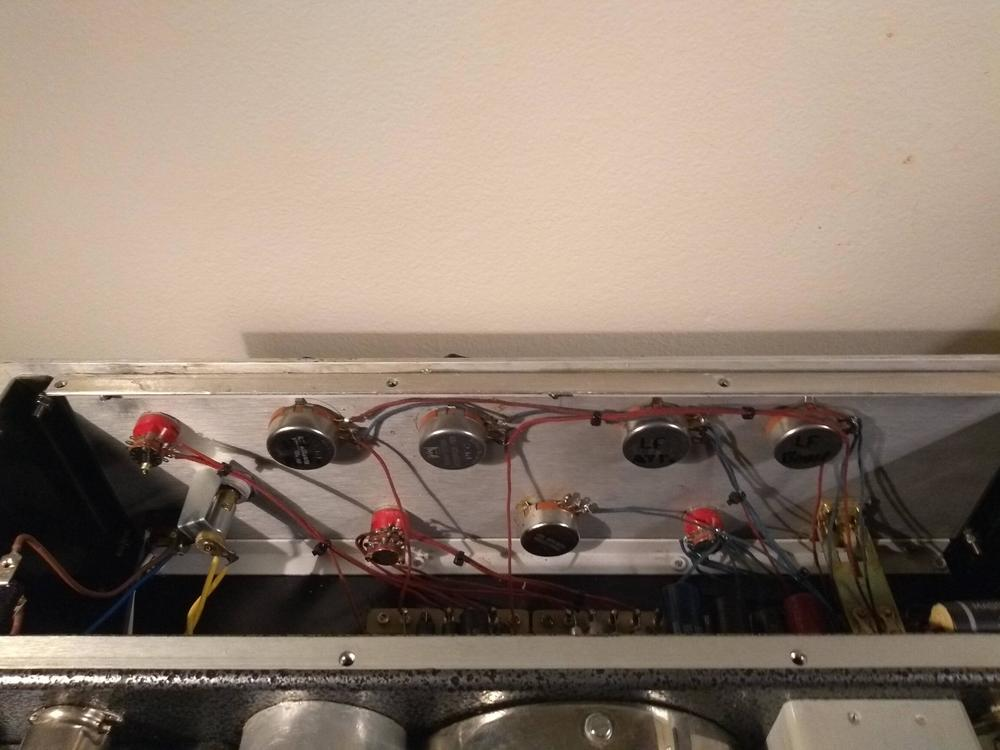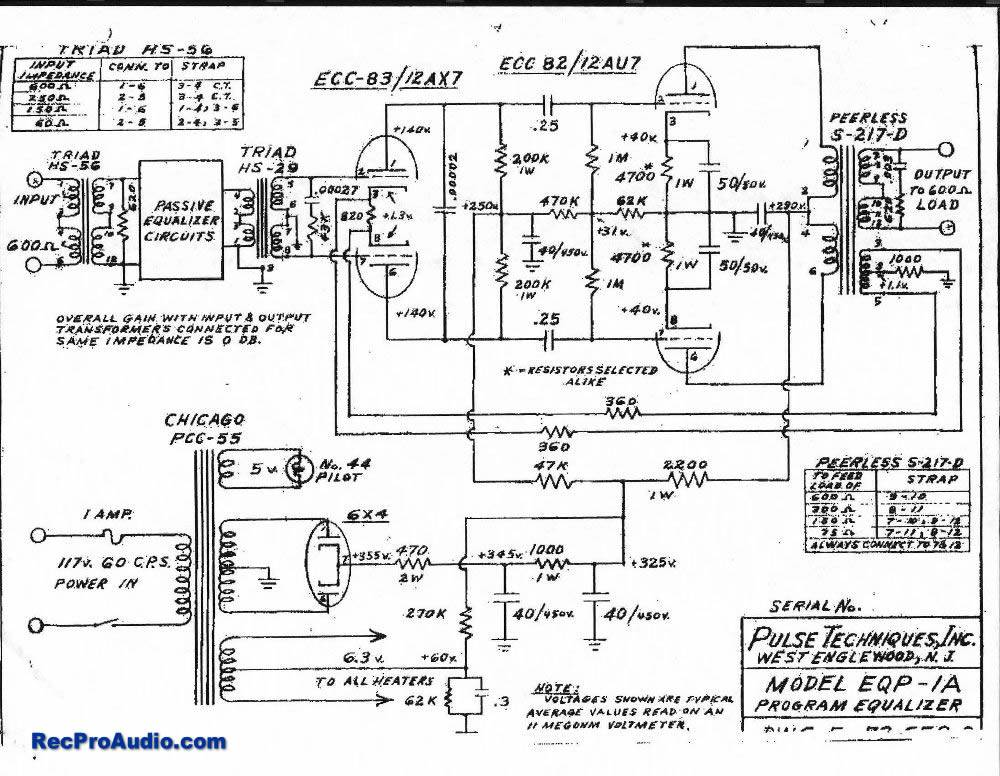Perhaps this is demonstrating my ignorance, but were are the inductors in the unit in the pictures?